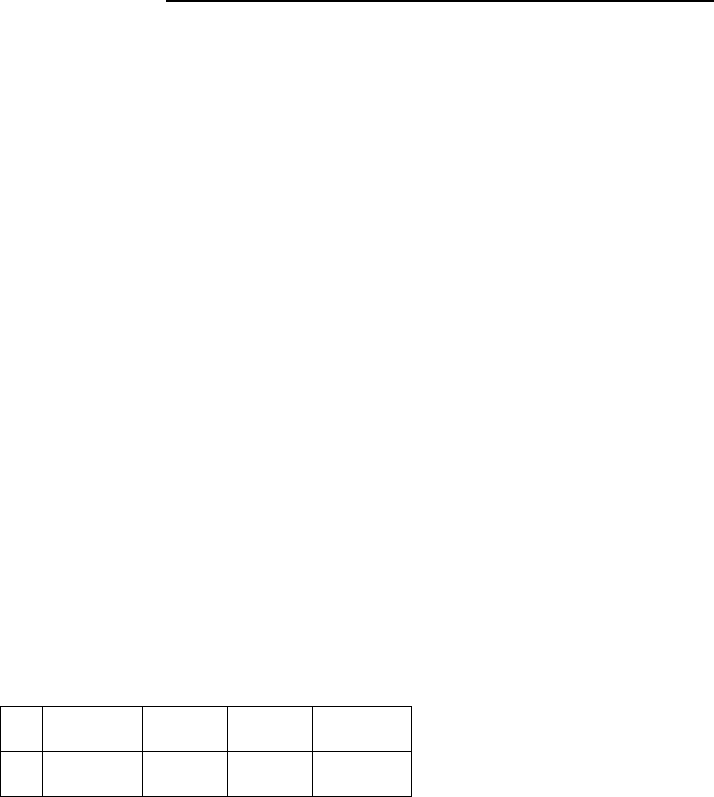# CHEM 1040 Lecture Notes - Lecture 27: Equivalence Point, Chief Operating Officer, Titration

68 views4 pages
School
Department
Course
ProfessorCHEM 1040 Lecture 27 Buffer Titrations and Graphs
Equivalence pH
The pH at equivalence point can be calculated by putting the salt produced through
neutralization in water
EX: For CH3COOH/NaOH
The neutralization process is:
CH3COOH + OH- CH3COO- + H2O
The moles of all species are equal. Supposing the concentration of CH3COOH and OH-
are both 0.10mol/L, and you have 0.05L of each, the concentration of CH3COO- will be
given by;
[CH3COO-] = (0.050*0.10) / 0.10 = 0.050mol/L
The equilibrium process to solve for pH is then the salt produced in water:
CH3COO- + H2O CH3COOH + OH-
I
0.050
0
0
C
0.050-x
x
x
KCH3COO- = Kw/KCH3COOH = 1.0E-14/1.8E-5 = 5.6E-10 = x2/0.050
X = 5.6E-10*0.050 = 5.3E-6 = [OH-]
Once the concentration of OH- is found, you can calculate the pH:
pH = 14 + log[OH-] = 8.73
Choosing an Indicator
The choice of indicator depends on:
pH at equivalence
pH at the end point of the indicator
Unlock document

This preview shows page 1 of the document.
Unlock all 4 pages and 3 million more documents.

## Document Summary

Chem 1040 lecture 27 buffer titrations and graphs. The ph at equivalence point can be calculated by putting the salt produced through neutralization in water. Supposing the concentration of ch3cooh and oh- are both 0. 10mol/l, and you have 0. 05l of each, the concentration of ch3coo- will be given by; The equilibrium process to solve for ph is then the salt produced in water: Kch3coo- = kw/kch3cooh = 1. 0e-14/1. 8e-5 = 5. 6e-10 = x2/0. 050. Once the concentration of oh- is found, you can calculate the ph: ph = 14 + log[oh-] = 8. 73. The choice of indicator depends on: ph at equivalence, ph at the end point of the indicator. For the best choice of indicator, you want these values to be as equal as possible. After equivalence: after equivalence has been reached, you must calculate the excess of the strong titrant. Excess reagent is oh- = 0. 0051 0. 0050 = 0. 00010mol of excess.

# Get access

\$10 USD/m
Billed \$120 USD annually
Homework Help
Class Notes
Textbook Notes